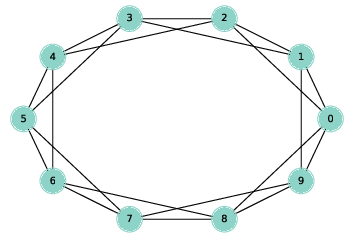# 5.4. Ring lattice¶Figure 5.1: A ring lattice with $$n=10$$ and $$k=4$$.

A regular graph is a graph where each node has the same number of neighbors; the number of neighbors is also called the degree of the node.

A ring lattice is a kind of regular graph, which Watts and Strogatz use as the basis of their model. In a ring lattice with $$n$$ nodes, the nodes can be arranged in a circle with each node connected to the $$k$$ nearest neighbors.

For example, a ring lattice with $$n=3$$ and $$k=2$$ would contain the following edges: $$(0, 1)$$, $$(1, 2)$$, and $$(2, 0)$$. Notice that the edges “wrap around” from the highest-numbered node back to $$0$$.

More generally, we can enumerate the edges like this:

def adjacent_edges(nodes, halfk):
n = len(nodes)
for i, u in enumerate(nodes):
for j in range(i+1, i+halfk+1):
v = nodes[j % n]
yield u, v


adjacent_edges takes a list of nodes and a parameter, halfk, which is half of $$k$$. It is a generator function that yields one edge at a time. It uses the modulus operator, $$\%$$, to wrap around from the highest-numbered node to the lowest.

We can test it like this:

>>> nodes = range(3)
>>> for edge in adjacent_edges(nodes, 1):
...     print(edge)
(0, 1)
(1, 2)
(2, 0)


Now we can use adjacent_edges to make a ring lattice:

def make_ring_lattice(n, k):
G = nx.Graph()
nodes = range(n)

Notice that make_ring_lattice uses floor division to compute halfk, so it is only correct if k is even. If k is odd, floor division rounds down, so the result is a ring lattice with degree k-1. As one of the exercises at the end of the chapter, you will generate regular graphs with odd values of k.
We can test make_ring_lattice like this:
lattice = make_ring_lattice(10, 4)